Fully Modified Estimation with Nearly Integrated Regressors

NOTE: International Finance Discussion Papers are preliminary materials circulated to stimulate discussion and critical comment. References to International Finance Discussion Papers (other than an acknowledgment that the writer has had access to unpublished material) should be cleared with the author or authors. Recent IFDPs are available on the Web at http://www.federalreserve.gov/pubs/ifdp/. This paper can be downloaded without charge from the Social Science Research Network electronic library at http://www.ssrn.com/.

Abstract:

I show that the test procedure derived by Campbell and Yogo (2005, Journal of Financial Economics, forthcoming) for regressions with nearly integrated variables can be interpreted as the natural t-test resulting from a fully modified estimation with near-unit-root regressors. This clearly establishes the methods of Campbell and Yogo as an extension of previous unit-root results.

JEL classification: C22

Keywords: Fully modified estimation; Near-unit-roots; Predictive regressions.

1  Introduction

In the recent past, there has been much effort spent on the econometric analysis of forecasting regressions with nearly persistent regressors. Using Monte Carlo simulations, Mankiw and Shapiro (1986) showed in an influential paper that when the regressor variables in a predictive regression are almost persistent and endogenous, test statistics will no longer have standard distributions. Since then, a much better understanding of this phenomenon has been established and several alternative methods have been proposed; e.g. Cavanagh et al. (1995), Stambaugh (1999), Jansson and Moreira (2004), Lewellen (2004), and Campbell and Yogo (2005).

The issues encountered when performing inference in forecasting regressions with near-persistent variables are, of course, similar to those in the cointegration literature where the regressors are assumed to follow unit-root processes. Indeed, the case with nearly persistent regressors can be seen as a generalization of the standard unit-root setup.

In this note, I show that the efficient test for inference in predictive regressions derived by Campbell and Yogo (2005) can also be seen as the natural test resulting from a generalization of fully modified estimation (Phillips and Hansen, 1990, and Phillips, 1995) to the case of near-unit-root regressors. In addition, the optimality properties of the Campbell and Yogo (2005) test-statistic can be seen as a direct analogue of the optimal inference results derived by Phillips (1991) for cointegrated unit-root systems. These results firmly establish the link between current work on predictive regressions and earlier work on cointegration between unit-root variables.

2  Model and assumptions

Let the dependent variable be denoted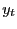, and the corresponding vector of regressors,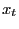, whereis an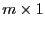vector and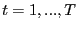. The behavior ofandare assumed to satisfy,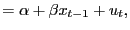(1)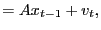(2)

where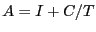is an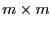matrix.
Assumption 1   Assumption 1 Let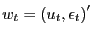and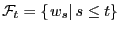be the filtration generated by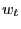. Then

1.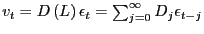, and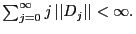2.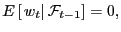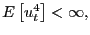and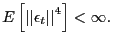3.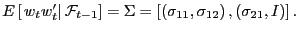The model described by equations (1) and (2) and Assumption 1 captures the essential features of a predictive regression with nearly persistent regressors. It states the usual martingale difference (mds) assumption for the errors in the dependent variables but allows for a linear time-series structure in the errors of the predictors. The error terms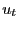and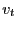are also often highly correlated. The auto-regressive roots of the regressors are parametrized as being local-to-unity, which captures the near-unit-root behavior of many predictor variables, but is less restrictive than a pure unit-root assumption.

The local-to-unity parameter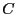is generally unknown and not consistently estimable. Following Campbell and Yogo (2005), I derive the results under the assumption thatis known. Bonferroni type methods can then be used to form feasible tests, as in Cavanagh et al. (1995) and Campbell and Yogo (2005); such methods are extensively explored in these papers and will not be further discussed here.

Let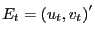be the joint innovations process. Under Assumption 1, by standard arguments,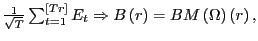where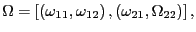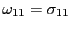,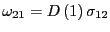,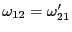,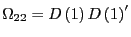, and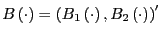denotes an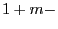dimensional Brownian motion. Also, let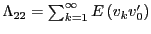be the one-sided long-run variance of. The following lemma sums up the key asymptotic results for the nearly integrated model in this paper (Phillips 1987, 1988).

Lemma 1   Lemma 1 Under Assumption 1, as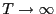, (a)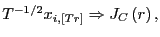(b)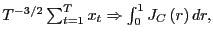(c)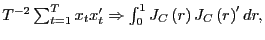(d)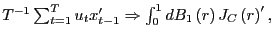and (e)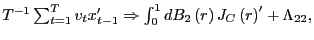where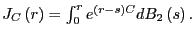Analogous results hold for the demeaned variables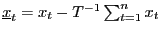, with the limiting process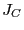replaced by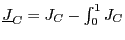.

3  Fully modified estimation

Let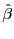denote the standard OLS estimate of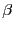in equation (1). By Lemma 1 and the continuous mapping theorem (CMT), it follows that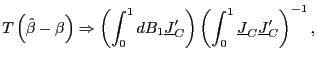(3)

as. Analogous to the case with pure unit-root regressors, the OLS estimator does not have an asymptotically mixed normal distribution due to the correlation between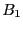and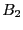, which causesandto be correlated. Therefore, standard test procedures cannot be used.

In the pure unit-root case, one popular inferential approach is to  fully modify'' the OLS estimator as suggested by Phillips and Hansen (1990) and Phillips (1995). In the near-unit-root case, a similar method can be considered. Define the quasi-differencing operator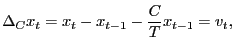(4)

and let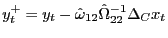and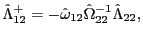where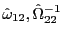, and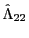are consistent estimates of the respective parameters.2 The fully modified OLS estimator is now given by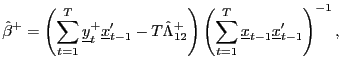(5)

where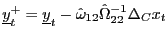and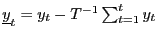. The only difference in the definition of (5), to the FM-OLS estimator for the pure unit-root case, is the use of the quasi-differencing operator, as opposed to the standard differencing operator. Once the innovationsare obtained from quasi-differencing, the modification proceeds in exactly the same manner as in the unit-root case.

Define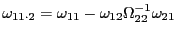and the Brownian motion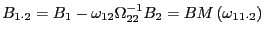. The process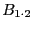is now orthogonal toand. Using the same arguments as Phillips (1995), it follows that, as,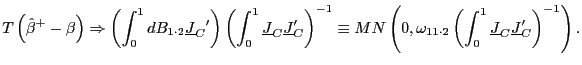(6)

The corresponding test-statistics will now have standard distributions asymptotically. For instance, the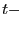test of the null hypothesissatisfies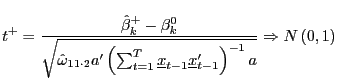(7)

under the null, as. Here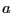is anvector with the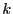'th component equal to one and zero elsewhere.

The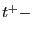statistic is identical to the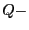statistic of Campbell and Yogo (2005). Whereas Campbell and Yogo (2005) attack the problem from a test point-of-view, the derivation in this paper starts with the estimation problem and delivers the test-statistic as an immediate consequence. However, presenting the derivation in this manner makes clear that this approach is a generalization of fully modified estimation.

In addition, if Assumption 1 is replaced by the stronger condition that bothandare martingale difference sequences, it is easy to show that OLS estimation of the augmented regression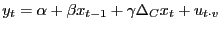(8)

yields an estimator ofwith an asymptotic distribution identical to that of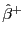. This is, of course, a straightforward extension of the results in Phillips (1991) for unit-root regressors. Moreover, in the unit-root case, Phillips (1991) shows that the OLS estimator ofin equation (8) is identical to the gaussian full system maximum likelihood estimator of. The optimality properties of Campbell and Yogo's (2005)test is thus a direct extension of the optimality results developed in Phillips (1991).

References

1. Campbell, J.Y., and M. Yogo, 2005. Efficient Tests of Stock Return Predictability, forthcoming Journal of Financial Economics.

2. Cavanagh, C., G. Ellliot, and J. Stock, 1995. Inference in models with nearly integrated regressors, Econometric Theory 11, 1131-1147.

3. Jansson, M., and M.J. Moreira, 2004. Optimal Inference in Regression Models with Nearly Integrated Regressors, NBER Working Paper T0303.

4. Lewellen, J., 2004. Predicting returns with financial ratios, Journal of Financial Economics, 74, 209-235.

5. Mankiw, N.G., and M.D. Shapiro, 1986. Do we reject too often? Small sample properties of tests of rational expectations models, Economic Letters 20, 139-145.

6. Phillips, P.C.B, 1987. Towards a Unified Asymptotic Theory of Autoregression, Biometrika 74, 535-547.

7. Phillips, P.C.B, 1988. Regression Theory for Near-Integrated Time Series, Econometrica 56, 1021-1043.

8. Phillips, P.C.B, 1991. Optimal Inference in Cointegrated Systems, Econometrica 59, 283-306.

9. Phillips, P.C.B, 1995. Fully Modified Least Squares and Vector Autoregression, Econometrica 63, 1023-1078.

10. Phillips, P.C.B, and B. Hansen, 1990. Statistical Inference in Instrumental Variables Regression with I(1) Processes, Review of Economic Studies 57, 99-125.

11. Stambaugh, R., 1999. Predictive regressions, Journal of Financial Economics 54, 375-421.

Footnotes

1.  Tel.: +1-202-452-2436; fax: +1-202-263-4850; email: erik.hjalmarsson@frb.gov. The views presented in this paper are solely those of the author and do not represent those of the Federal Reserve Board or its staff. Return to text

2.  The definition of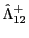is slightly different from the one found in Phillips (1995). This is due to the predictive nature of the regression equation (1), and the martingale difference sequence assumption onReturn to text